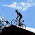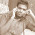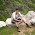## August 19, 2012

### Puzzle: Divide by 3 Counter with 50% DC

It is pretty simple to make a clock divider with odd frequency division (let's say 3 or 5). But it doesn't have 50% duty cycle. Some modifications are essential to achieve that 50% duty cycle. You might argue, why so much fuss about 50%? To give you an insight into it, consider the following divided waveform with 66% DC:

As you can note from the above waveforms:
• NEG-TO-POS arc (i.e. any path launching from a negative edge triggered flop and being captured at positive edge triggered flop) would have least time to meet the setup time requirement and hence can be critical.
• On the other hand, POS-TO-POS and NEG-TO-NEG are so much relaxed.
Same would be true for a divider with 33% duty cycle as well. So, it is preferable to use a divided clock with 50% duty cycle.

Can you design such a circuit which takes a clock signal of frequency f, and outputs another clock signal of frequency f/3 with 50% duty cycle?

#### 8 comments:

1.Yes I can

1.Hello. Would really appreciate it if you can post or mail me a solution. Would love to share it with the readers.

Thanks!

2.It can be done by one pos-edge triggered state machine giving output as A=110 and another neg-edge triggered state machine giving output as B=011 and finally Y=A.B'

1.Would really appreciate it if you can mail me a solution.why you taking 110 and 011 input only
prashantguptaengg@gmail.com

3.Here is the code:
Note: clk3 = clk/3

`timescale 1ns/1ps
module clk3;
reg clk;
wire clk3;
reg clk110, clk011;

reg [1:0] ccnt110;
reg [1:0] ccnt011;

assign clk3 = clk110 & clk011;

initial begin
clk=0;
ccnt110=0;
ccnt011=0;
\$display("clk=%b ccnt110=%d ccnt011=%d", clk, ccnt110,ccnt011);
forever
begin #10
clk=~clk;
\$display("clk=%b ccnt110=%d ccnt011=%d", clk, ccnt110,ccnt011);
end
end

always@(posedge clk)
begin
ccnt110++;
if(ccnt110 == 2'd3) begin
ccnt110=2'd0;
clk110=0;
end else
clk110=1;
end

always@(negedge clk)
begin
ccnt011++;
if(ccnt011 == 2'd3) begin
ccnt011=2'd0;
clk011=0;
end else
clk011=1;
end

initial begin
#1000 \$finish;
end

//initial begin
//\$vcdpluson;
//end

endmodule

4.1. Design a divide by 3 counter (active on posedge of clock)
2. Give the LSB bit output of the counter(q0) to a D Flip flop acting on negedge of clock. (same clock as counter)
3. Give q0 and the output of DFF to an OR gate. The output of OR gate gives the divided by 3 clock with 50% dutycycle.

Thanks to : vlsiupdatez //refer this blog to see odd division of clock with 50% dutycycle

Regards,
Vaidhy.

1.Thank you, dear Anonymous!

The purpose of this puzzle was to elicit the answer out of the readers and maybe start a discussion on which one is a better implementation based on the timing and power constraints.

Thanks, anyway for posting the answer.

2.use neg edge clock to get neg edge of divde by3... however its still not balanced.